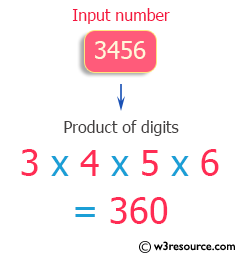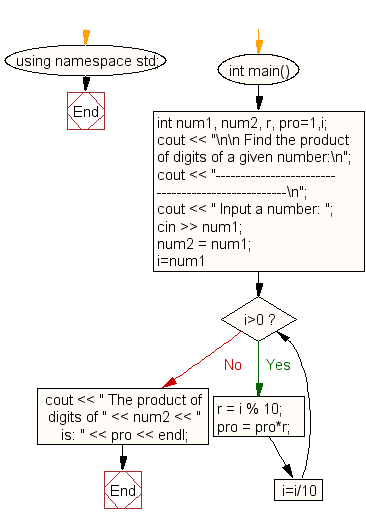﻿ C++ Exercises: Calculate product of digits of any number - w3resource

# C++ Exercises: Calculate product of digits of any number

## C++ For Loop: Exercise-58 with Solution

Write a program in C++ to calculate the product of the digits of any number.

Pictorial Presentation:Sample Solution:

C++ Code :

``````#include <iostream>
using namespace std;
int main()
{
int num1, num2, r, pro=1,i;
cout << "\n\n Find the product of digits of a given number:\n";
cout << "--------------------------------------------------\n";
cout << " Input a number: ";
cin >> num1;
num2 = num1;
for(i=num1;i>0;i=i/10)
{
r = i % 10;
pro = pro*r;
}
cout << " The product of digits of " << num2 << " is: " << pro << endl;
}
``````

Sample Output:

``` Find the product of digits of a given number:
--------------------------------------------------
Input a number: 3456
The product of digits of 3456 is: 360
```

Flowchart:C++ Code Editor: